# Deriving the speed of light from Maxwell’s equations

The speed of light in a vacuum is approximately 3,000,000 m/s (slightly less, in fact, but we will stick to this simpler number for now). There have been numerous experiments that have allowed us to measure this quantity very accurately, but there is a rather elegant way to arrive at the same number by using nothing more than mathematics and a few other less-complicated-to-measure numbers in physics. In this article, we will see how we can derive the speed of light (or at least a number sufficiently close to modern measurements) by using Maxwell’s equations of classical electromagnetism (warning: you will need to be familiar with vector calculus beforehand).

We will start our derivation with Maxwell’s equations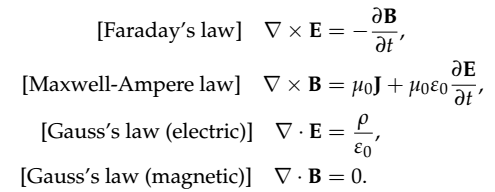Equation 1 (a) - (d)

If we confine ourselves to a vacuum, we can set the charge density 𝞺 = 0 and the current density J = 0. This leads to the simplified equations in terms of the electric field E and magnetic field B only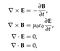Equation 2 (a) - (d)

Next, we could like to decouple the fields from each other, which can be done by applying the curl operator to both sides of Eq(2a) and Eq(2b)

Using the double-curl identity of vector calculus

we can write the left-hand side of our electromagnetic equations as

where the divergence terms are zero as a result of Eq(2c) and Eq(2d). Therefore the equations reduce to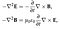Equation 3 (a) - (b)

Now, substituting Eq(2b) into Eq(3a) and Eq(2a) into Eq(3b) yields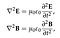Equation 4 (a) - (b)

Looking closely are these equations, we note that they look suspiciously similar to the more general wave equation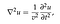Equation 5: the scalar wave equation

such that we can write

by setting the speed of light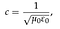Equation 6: speed of light in a vacuum

where 𝝻0 = 4𝝿 𝗑 10^(-7) H/m (permeability of free space) and ε0 = 8.85418782 × 10^(-12) m^(-3) kg^(-1) s^4 A^2 (permittivity of free space). Putting these values into Eq(6) yields the estimate for the speed of light in a vacuum

c = 2,997,925 m/s.

This result demonstrates two very important points: first, it tells us that Maxwell’s equations in a vacuum can be solved in terms of waves, and secondly that both magnetic and electric fields propagate at exactly the same speed c. We can alternatively look at these equations in frequency space by taking the temporal Fourier transform of both sides to yield

from which we obtain the wavenumber k = ω/c, reducing our expressions to a set of Helmholtz equations

The solution to Maxwell’s equations in a vacuum admits any travelling waves of the type f(r - ct). One particular case of interest involves time-harmonic fields, for which we can use the solutions

where the wavevector obeys the relation |k| = ω/c and c.c. denotes the complex conjugate of the first term (this is because the electric and magnetic fields are real quantities, not to confuse c.c. with the speed of light). The ratio of the magnitudes of the two waves is therefore

To figure out what this constant is, it suffices to invoke the Lorentz force acting on a charge q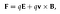Equation 7: the Lorentz force

and assuming a constant speed v with no other external forces, such that F = 0 we obtain

Thus, taking the magnitude of both sides yields

Furthermore, in the special case where the angle θ = 𝛑/2 (or 90 degrees, implying the two fields are perpendicular) we obtain

This means that the ratio of the magnitudes between the electric and magnetic fields in a vacuum must be equal to the speed of propagation of the waves. Therefore, one can easily re-write the two solutions in terms of the same field amplitude E0

Oscar is a physicist, educator and STEM enthusiast. He is currently finishing a PhD in Theoretical Physics with a focus on photonics and stochastic dynamics.

## More from Oscar Nieves

Oscar is a physicist, educator and STEM enthusiast. He is currently finishing a PhD in Theoretical Physics with a focus on photonics and stochastic dynamics.